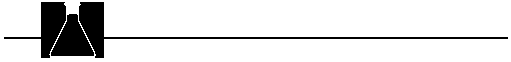## Group and Discussion Activities

1. Why do chemists often refer to moles of a substance rather than molecules (or atoms or formula units)? [The mole is a convenient size unit to express the amounts of substances involved in laboratory-scale reactions.]
2. How are moles, mass, and number of entities for a sample of matter related? [1 mol of entities = formula mass (in grams) x (the molar mass) = 6.02 x 1023 entities]
3. What is the relationship of moles to volume of a gas at STP? [For an ideal gas at STP, 1 mol = 22.4 L ]
4. How is percent composition related to mole ratios? [The percent of an element (A) in a compound is given by:5. How is the empirical formula related to the mole ratios of elements in a compound? [The empirical formula relates to the relative numbers of moles of each element in the compound. The relative numbers of moles expressed as integers are the subscripts in the empirical formula. For example, if a compound contains 1.5 mol A for every mole of B, the ratio expressed as integers is 3:2-the empirical formula for the compound is A3B2 .]
6. Explain why equal masses of different substances do not contain equal numbers of molecules or moles. [Since different substances usually do not have the same molar mass, one mole or Avogadro's number of entities (molecules, etc.) will have different masses. Therefore, equal masses must contain different numbers of moles or molecules.]

1. 200 g of lead has fewer atoms than 1 g of hydrogen. (Other examples can be given.) One mole of lead (208 g) has the same number of atoms (Avogadro's number, or 6.02 x 1023 ) as one mole of hydrogen (1 g). However, 200 g of lead is less than one mole of lead and has fewer than Avogadro's number of atoms, thus fewer than 1 g of hydrogen.